This in in continuation to the last blog post in which we talked about the basics, separable type and linear type differential equation. If you haven’t read it already, do check it out here:

What is a differential equation?

We will talk about another type of ODE called exact differential equation

If the differential equation of the typecan be written in the form of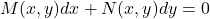andthen this form is called exact. The following examples clarifies the procedure to solve:

For instance,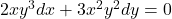Now, We must find a functionsuch thatThen, the solution would be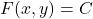, where C is an arbitrary constant

I have covered some questions on this in the below video.

Another important type is Homogenous linear differential equation

It involves only derivatives of y and terms involving y, and they’re set to 0, as in this equation:This equation is then converted into a characteristic equation: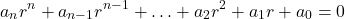Depending on the roots (value of r) of this equation, we find the solution.

We would consider the case of a 2nd order homogenous linear equation.

Case1: If both the roots are real and distinctand, then solution is:where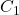and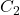are arbitrary real numbers.

Case2: If both the roots are real and equal, then solution is:Case3: If both the roots are complex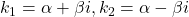, then solution is:I have covered various questions on these two types in this video.